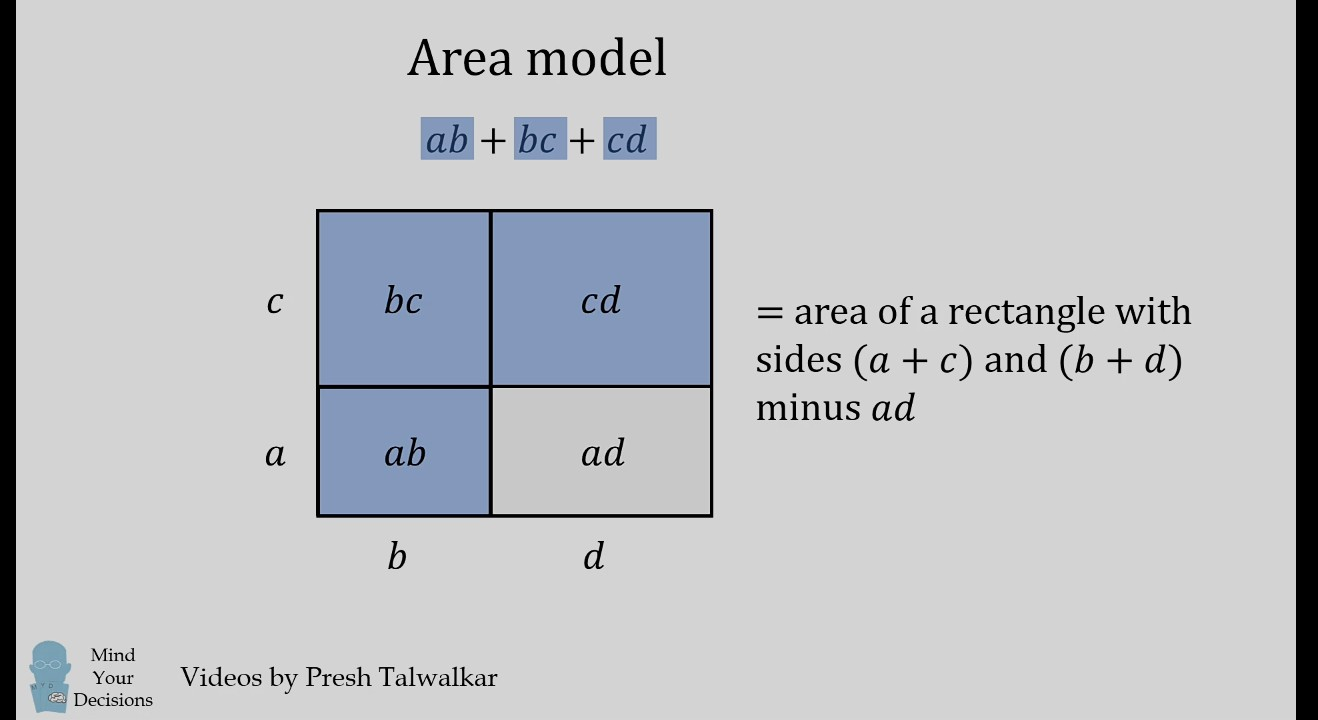# TIL (today I learned)

• you can express product of two numbers (x,y) as the area of a rectangle with side x and y, which may make it easier to solve like this.• when you spilt a number x in x/2 and x/2, and then multiply them, you get the highest product than any other slipts, for eg.
10 -> 5 * 5 = 25 will be biggest
6 * 4 = 24
7 * 3 = 21
8 * 2 = 16
9 * 1 = 9